# Similar Figures Similar Similar figures have the same

• Slides: 11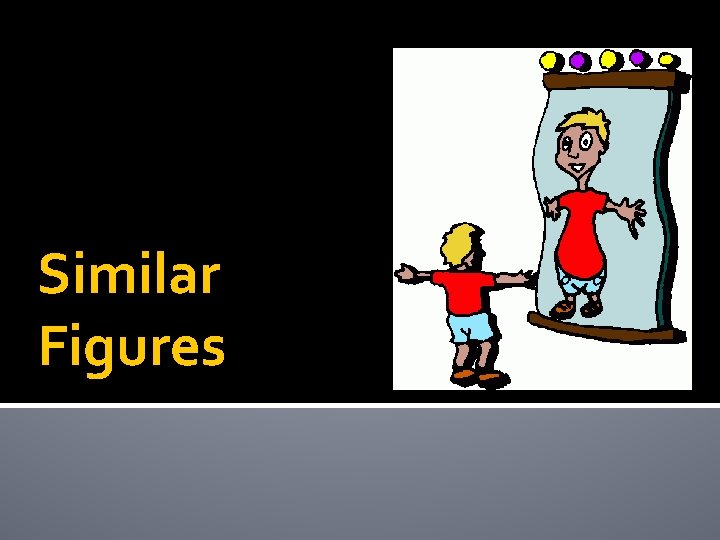Similar FiguresSimilar �Similar figures have the same shape, but not necessarily the same size.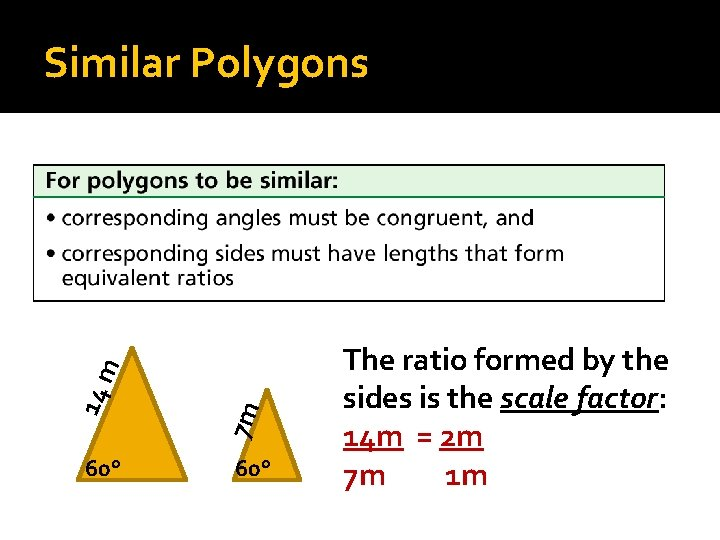60° 7 m 14 m Similar Polygons 60° The ratio formed by the sides is the scale factor: 14 m = 2 m 7 m 1 mUse proportions to find the length of the missing side of a similar polygon. A 1. 2. 3. 4. 5. P m B c 15 12 cm N X M m 5 c ∆ABC is similar to ∆NMP. Find the length of X. C Set up a proportion: Large ∆ to Small ∆ Large ∆ 15 = 12 Small ∆ 5 x Cross multiply and solve. 15 x = 60 x=4 The missing length of ∆NMP is 4 cm.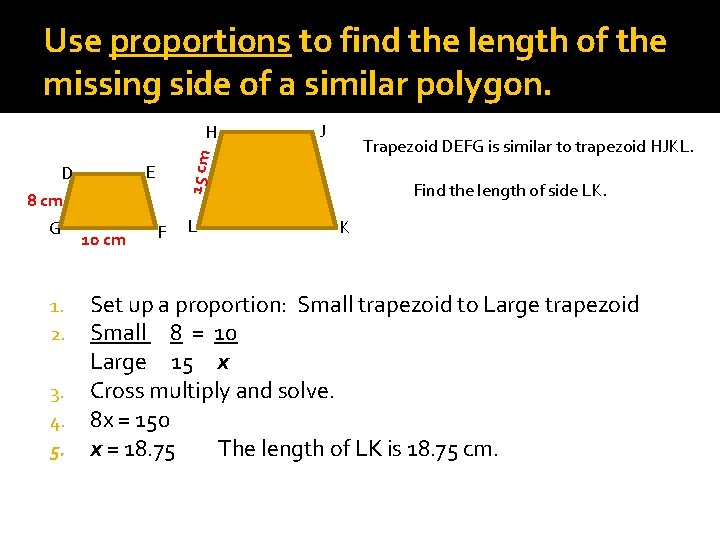Use proportions to find the length of the missing side of a similar polygon. E D 8 cm G 1. 2. 3. 4. 5. 10 cm J Trapezoid DEFG is similar to trapezoid HJKL. 15 cm H F L Find the length of side LK. K Set up a proportion: Small trapezoid to Large trapezoid Small 8 = 10 Large 15 x Cross multiply and solve. 8 x = 150 x = 18. 75 The length of LK is 18. 75 cm.Use proportions to find the length of the missing side of a similar polygon. F 1. 2. 3. 4. 5. 6. 6 m B R S 8 m 20 m A C 12 m D Polygon ABCDEF is similar to polygon RSTUVW. Find the lengths of RS and WV. TU W V E Set up a proportion: Large polygon to Small polygon Large 20 = 6 Small 8 x (RS) Cross multiply and solve. 20 x = 48 x = 2. 4 The length of RS is 2. 4 m. 1. 2. 3. 4. 5. 6. Set up a proportion: Large polygon to Small polygon Large 20 = 12 Small 8 x (WV) Cross multiply and solve. 20 x = 96 x = 4. 8 The length of WV is 4. 8 m.Use Scale Factors to Find Missing Dimensions A picture 10 in. tall and 14 in. wide is to be scaled to 1. 5 in. tall to be displayed on a Web page. How wide should the picture be on the Web page for the two pictures to be similar? Step 1: Draw & Label a picture. Step 2: Set up a proportion. Large 10 = 14 Small 1. 5 x 14(1. 5) = 10 x 21 = 10 x 1. 5 in. 10 in. 14 in. ? in. The picture should be 2. 1 in. wide.Use Scale Factors to Find Missing Dimensions A flag in the shape of an isosceles triangle with side lengths 18 ft, and 24 ft is hanging on a pole outside a campground. A camp t-shirt shows a smaller version of the triangle with two sides that are each 4 in. long. What is the length of the third 24 ft side of the triangle on the t-shirt? Set up a proportion. 18 ft = 4 in. 24 ft x in. 18 ft • x in. = 24 ft • 4 in. Find the cross products. 18 x = 96 Multiply x =96 5. 3 Solve for x. 18 The third side of the triangle is about 5. 3 in. long. 18 ft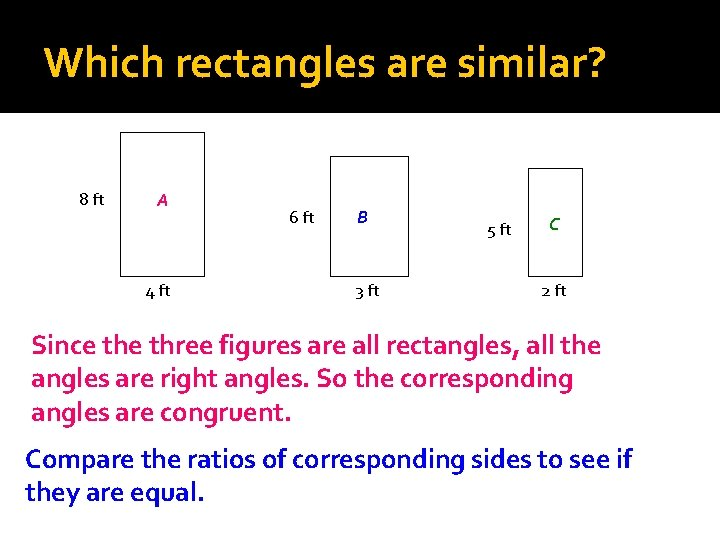Which rectangles are similar? 8 ft A 4 ft 6 ft B 3 ft 5 ft C 2 ft Since three figures are all rectangles, all the angles are right angles. So the corresponding angles are congruent. Compare the ratios of corresponding sides to see if they are equal.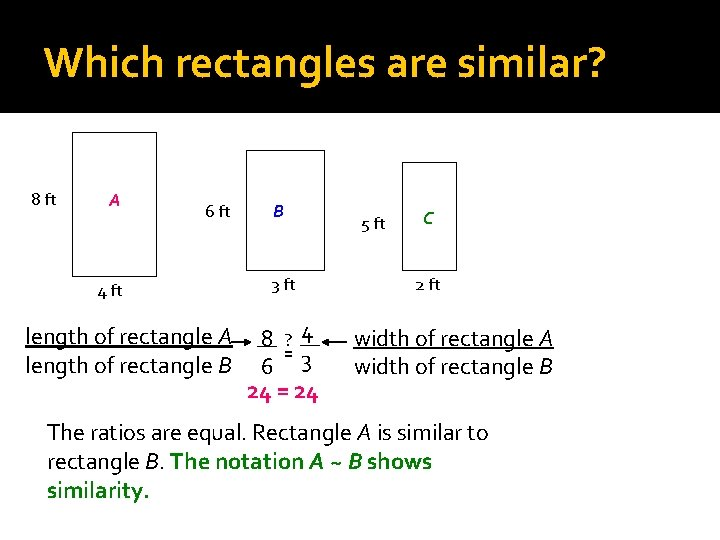Which rectangles are similar? 8 ft A 6 ft 4 ft length of rectangle A length of rectangle B B 3 ft 8 ? 4 = 6 3 24 = 24 5 ft C 2 ft width of rectangle A width of rectangle B The ratios are equal. Rectangle A is similar to rectangle B. The notation A ~ B shows similarity.Which rectangles are similar? 8 ft A 6 ft 4 ft length of rectangle A length of rectangle C B 3 ft 8 ? 4 = 5 2 16 20 5 ft C 2 ft width of rectangle A width of rectangle C The ratios are NOT equal. Rectangle A is NOT similar to rectangle C.